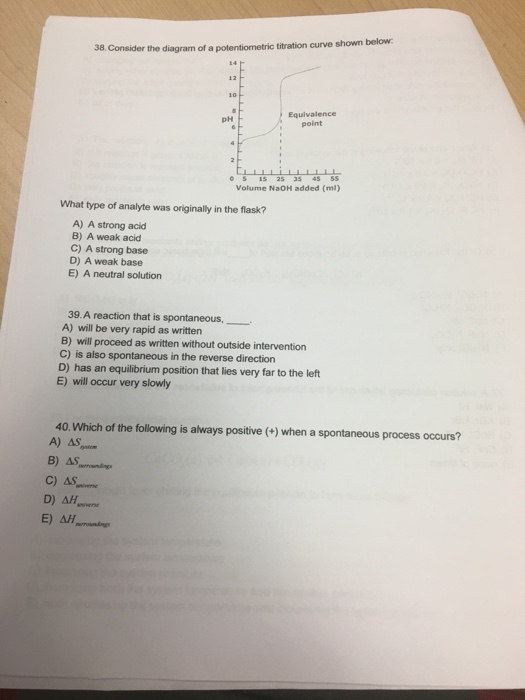# Question & Answer: Consider the diagram of a potentiometric titration curve shown below: What type of analyte…..Consider the diagram of a potentiometric titration curve shown below: What type of analyte was originally in the flask? A) A strong acid B) A weak acid C) A strong base D) A weak base E) A neutral solution A reaction that is spontaneous, _____ A) will be very rapid as written B) will proceed as written without outside intervention C) is also spontaneous in the reverse direction D) has an equilibrium position that lies very far to the left E) will occur very slowly Which of the following is always positive (+) when a spontaneous process occurs? A) Delta S_system B0 Delta surroundings C) Delta S_universe D) Delta H_universe E) Delta H_surroundings

Q38

This appears to have buffer region, in which addition of titrant does not changes pH drastically,

Don't use plagiarized sources. Get Your Custom Essay on
Question & Answer: Consider the diagram of a potentiometric titration curve shown below: What type of analyte…..
GET AN ESSAY WRITTEN FOR YOU FROM AS LOW AS \$13/PAGE

therefore, it must be a wek acid, since it is a low pH, but will form a buffer region

Q39.

a reaction that is spontaneous:

a) false, this is kinetics not equilbirium study

b) True, as if written is “forward” direction

c) false, it can’t be due to logic flow of events

d) false, this will be very reverse reaction

e) false, kinetics have nothing to do with equilibrium

choose B

Q40.

If a process is spontaneous

this implies that 2nd law of thermodynamics is being satisfied

which implies that the:

Total entropy of the UNIVERSE is increasing…

then

dSuniverse = positive